ConceptDraw Samples | Software Development — DOM Tree

# DOM Tree

The samples were created in ConceptDraw DIAGRAM using the DOM Tree Solution from ConceptDraw Solution Park. The ConceptDraw DIAGRAM offers you a variety of diagramming tools, and solutions from the Solution Park, provide diagram templates and libraries of ready-made charts and shapes for quick and simple drawing professional-quality business diagrams.

ConceptDraw DIAGRAM provides export of vector graphic multipage documents into multiple file formats: vector graphics (SVG, EMF, EPS), bitmap graphics (PNG, JPEG, GIF, BMP, TIFF), web documents (HTML, PDF), PowerPoint presentations (PPT), Adobe Flash (SWF).

## Tutorials and Solutions:

Video Tutorials: ConceptDraw Solution Park

Solutions: DOM Tree for ConceptDraw DIAGRAM

## Sample 1: DOM Hierarchy in HTML

DOM Tree sample: DOM Hierarchy in HTML.

This example is created using ConceptDraw DIAGRAM diagramming software enhanced with DOM Tree solution from ConceptDraw Solution Park.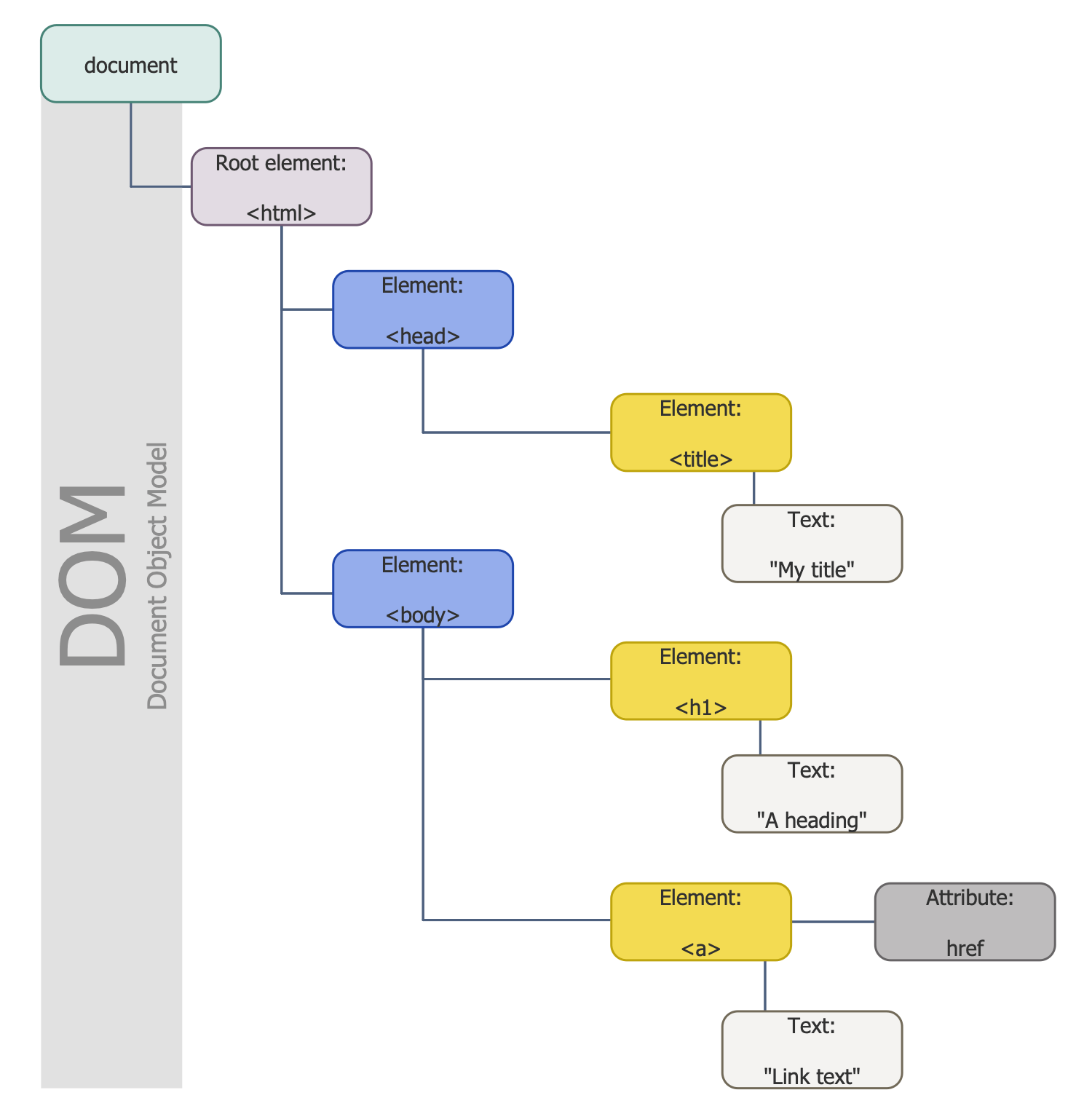This example is created using ConceptDraw DIAGRAM diagramming software enhanced with DOM Tree solution from ConceptDraw Solution Park.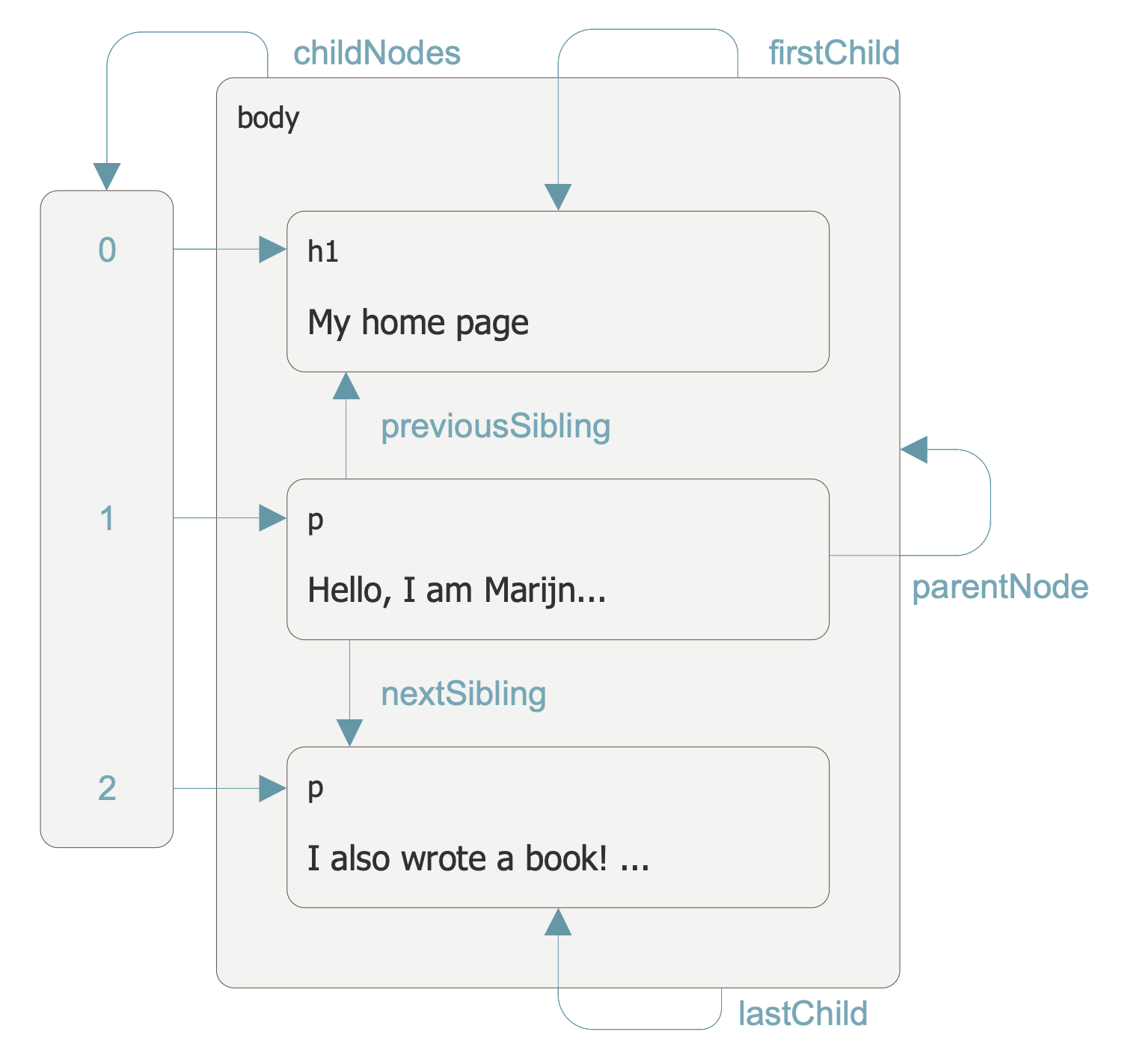## Sample 3: Dom Tree.

DOM Tree sample: Dom Tree.

This example is created using ConceptDraw DIAGRAM diagramming software enhanced with DOM Tree solution from ConceptDraw Solution Park.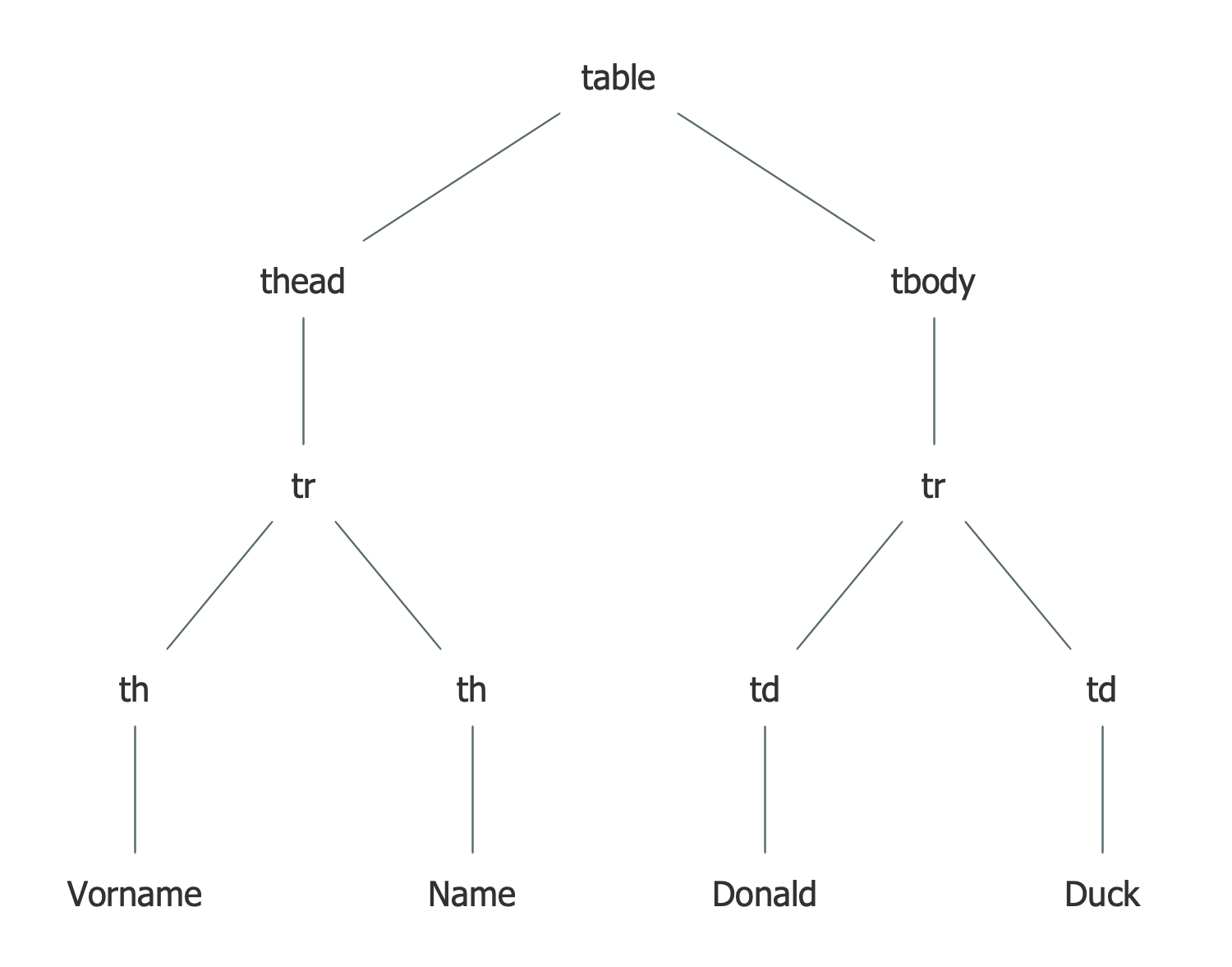## Sample 4: Dom Tree Mindmap

DOM Tree sample: Dom Tree Mindmap.

This example is created using ConceptDraw DIAGRAM diagramming software enhanced with DOM Tree solution from ConceptDraw Solution Park.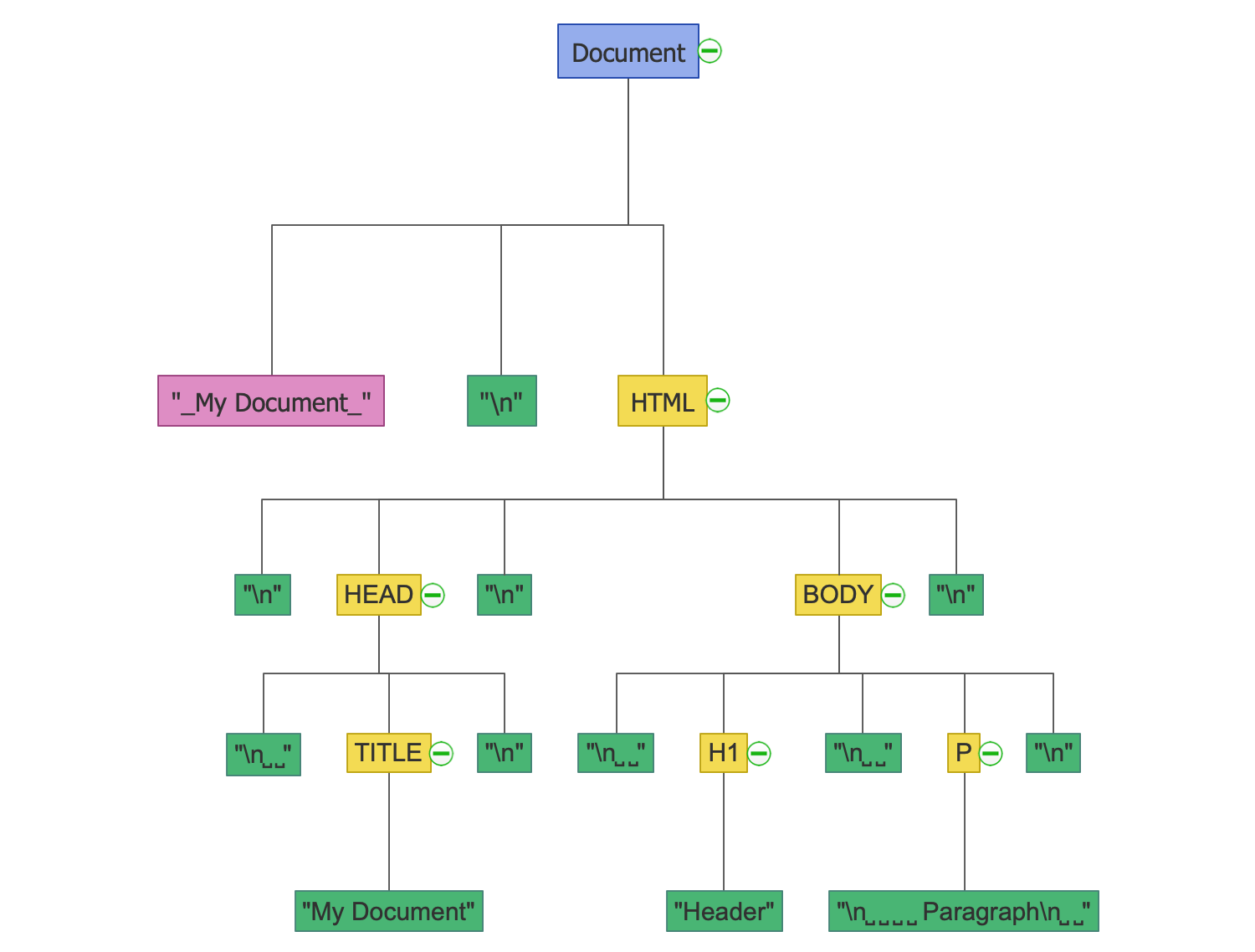## Sample 5: HTML Document Structure

DOM Tree sample: HTML Document Structure.

This example is created using ConceptDraw DIAGRAM diagramming software enhanced with DOM Tree solution from ConceptDraw Solution Park.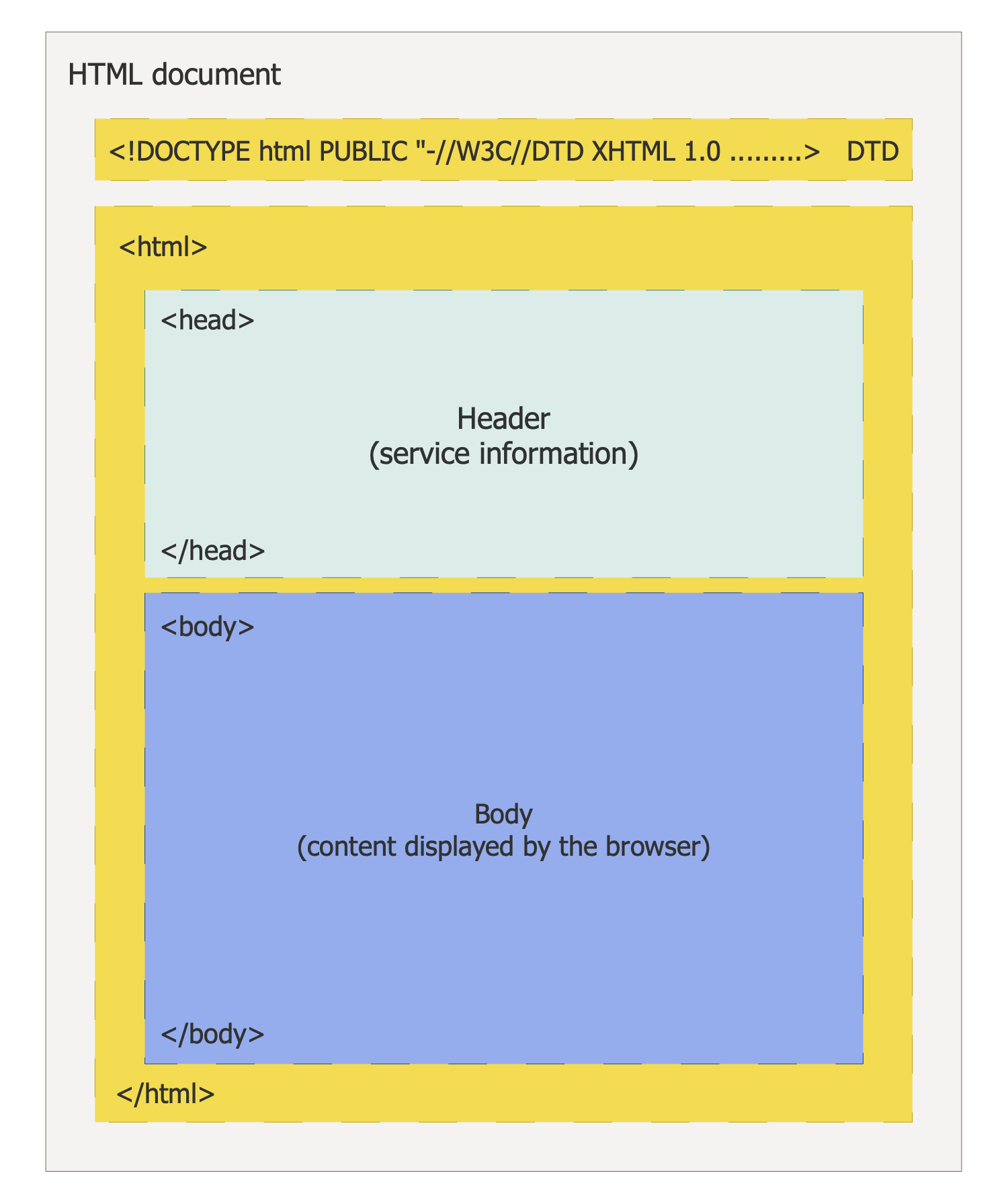## Sample 6: HTML DOM Mindmap

DOM Tree sample: HTML DOM Mindmap.

This example is created using ConceptDraw DIAGRAM diagramming software enhanced with DOM Tree solution from ConceptDraw Solution Park.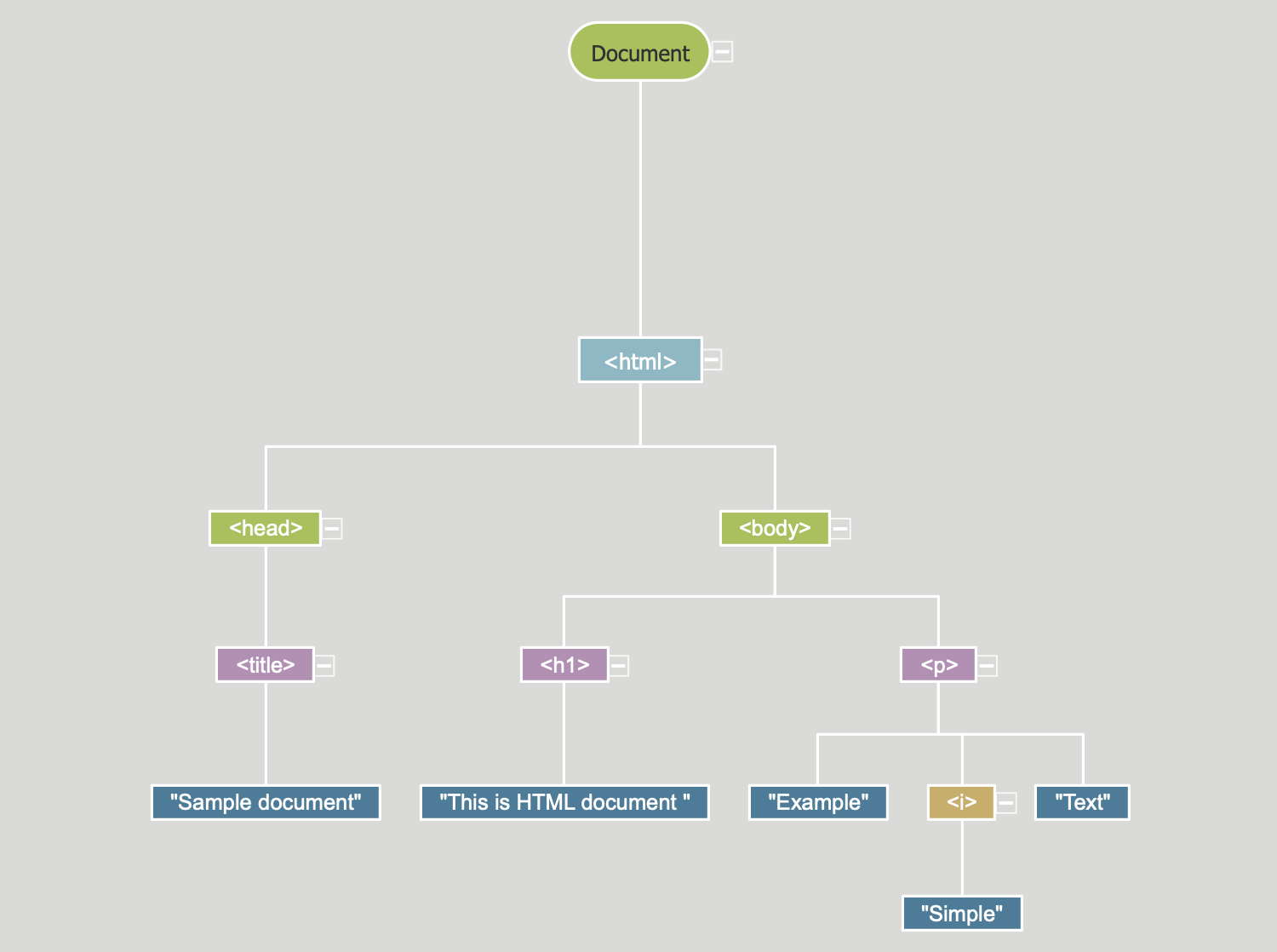## Sample 7: Objects Hierarchy in HTML DOM

DOM Tree sample: Objects Hierarchy in HTML DOM.

This example is created using ConceptDraw DIAGRAM diagramming software enhanced with DOM Tree solution from ConceptDraw Solution Park.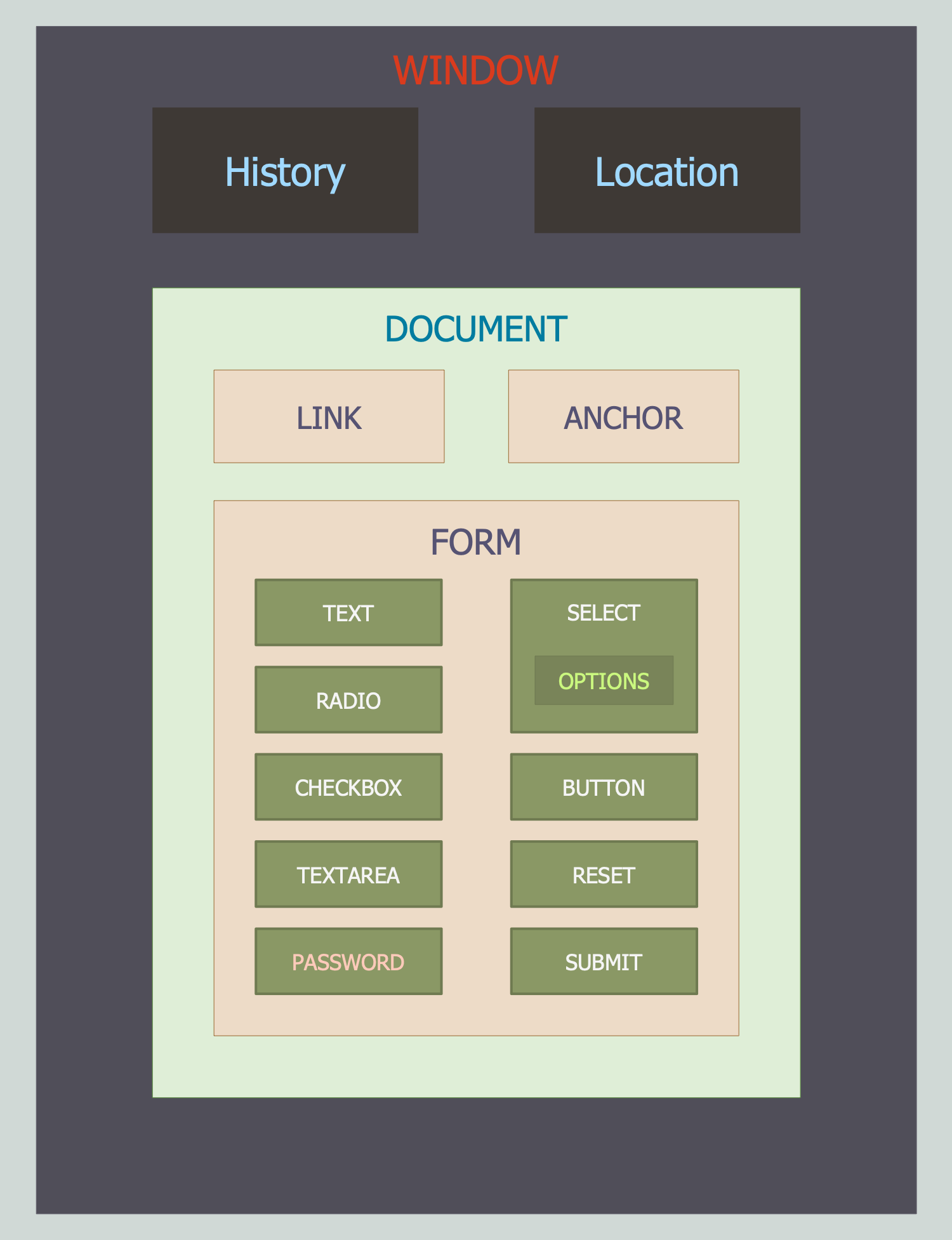## Sample 8: Simpe HTML Page DOM Mindmap

DOM Tree sample: Simpe HTML Page DOM Mindmap.

This example is created using ConceptDraw DIAGRAM diagramming software enhanced with DOM Tree solution from ConceptDraw Solution Park.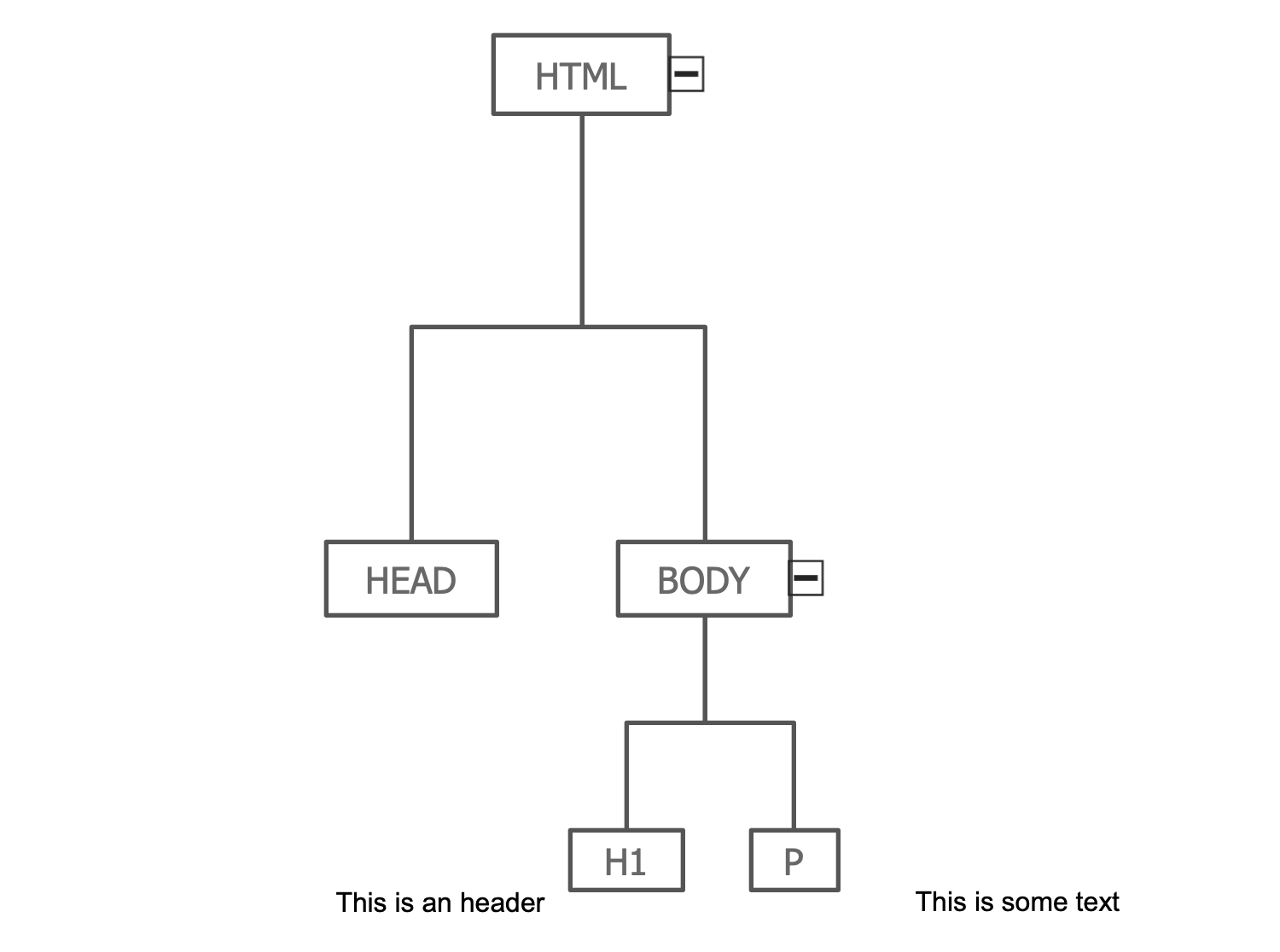## Sample 9: Web Page DOM

DOM Tree sample: Web Page DOM.

This example is created using ConceptDraw DIAGRAM diagramming software enhanced with DOM Tree solution from ConceptDraw Solution Park.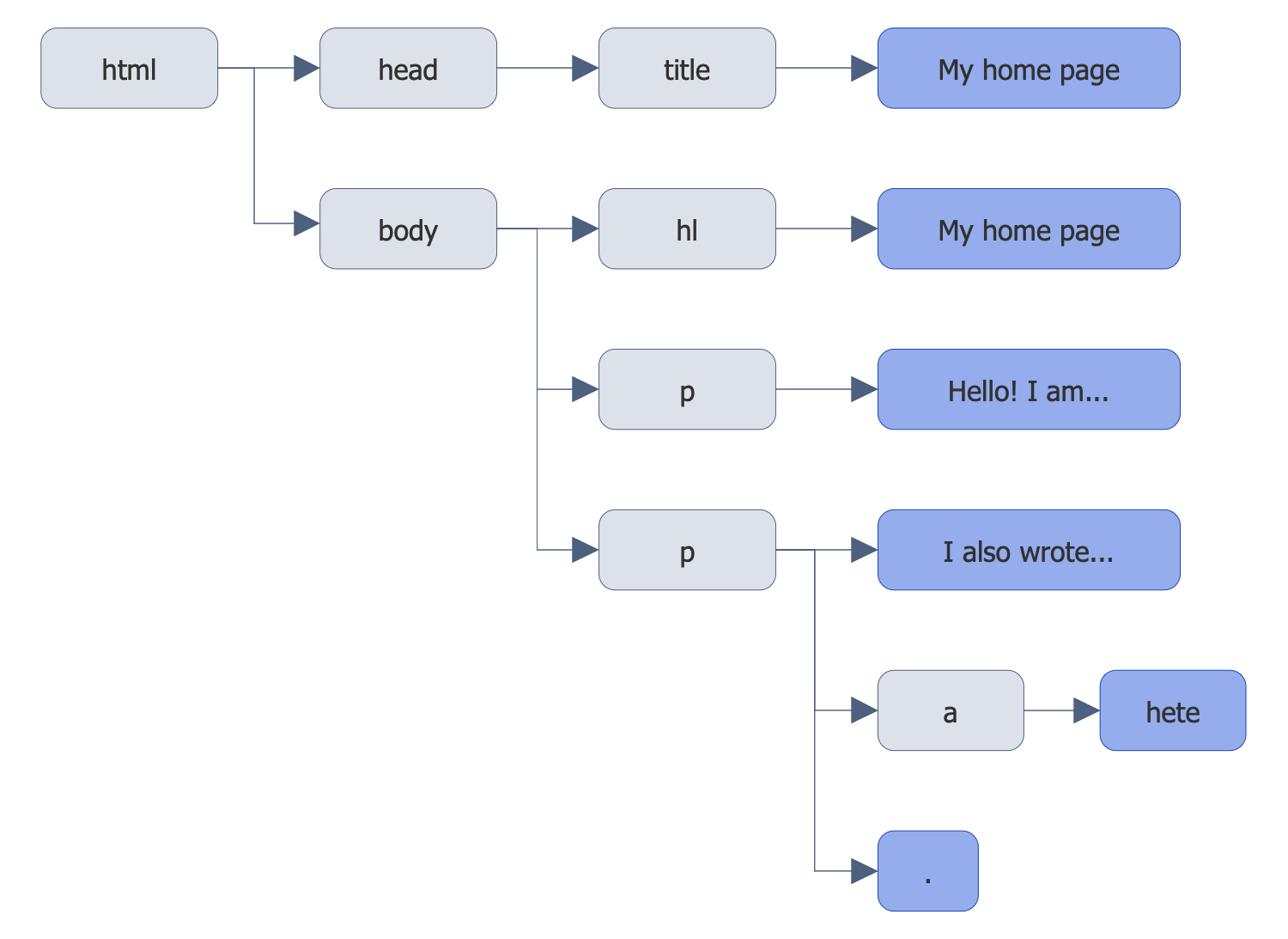## Sample 10: Web Page Structure

DOM Tree sample: Web Page Structure.

This example is created using ConceptDraw DIAGRAM diagramming software enhanced with DOM Tree solution from ConceptDraw Solution Park.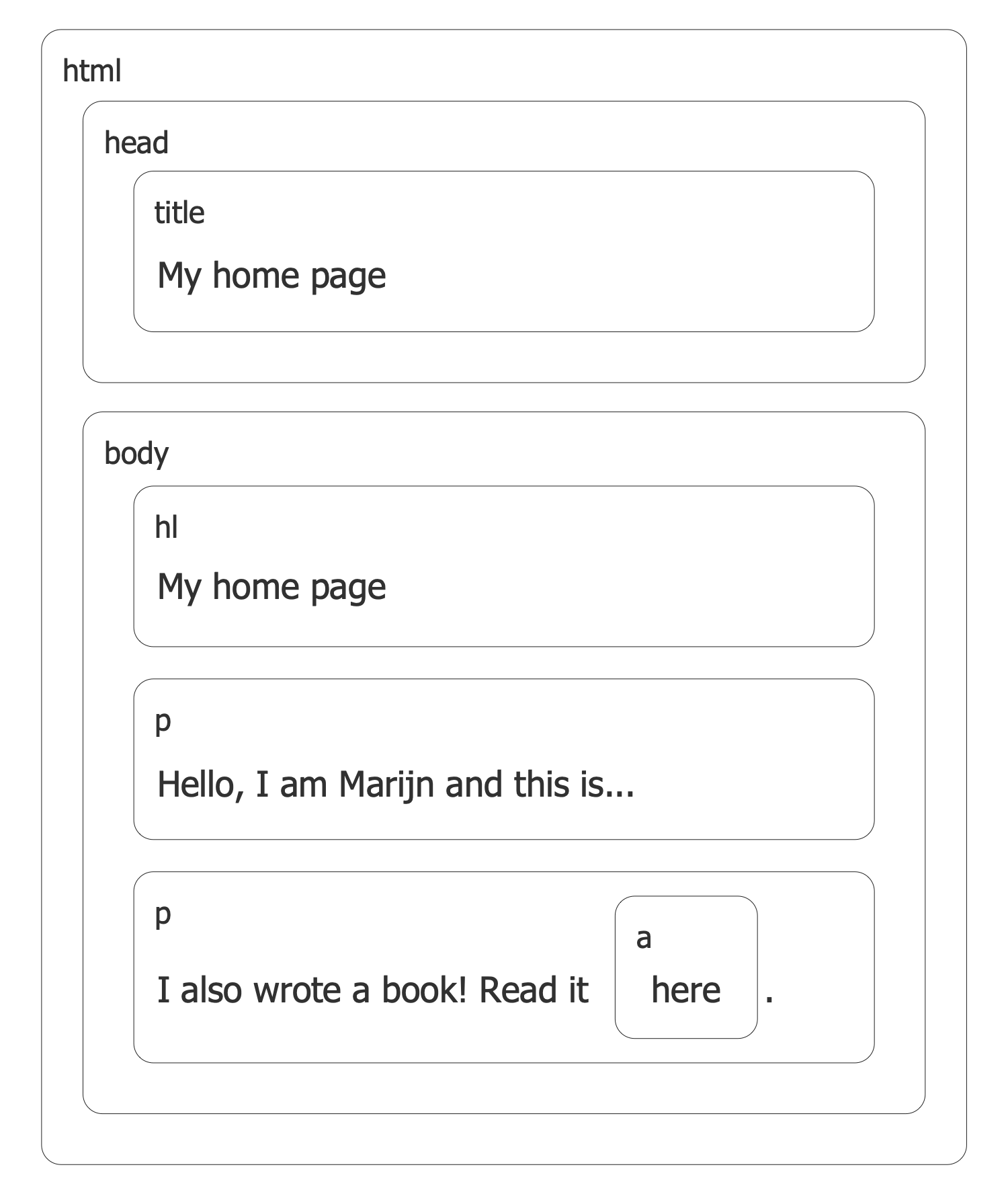All samples are copyrighted CS Odessa's.

Usage of them is covered by Creative Commons “Attribution Non-Commercial No Derivatives” License.

The text you can find at: https://creativecommons.org/licenses/by-nc-nd/3.0